Courses

# Test: Introduction To Complex Numbers

## 15 Questions MCQ Test Mathematics (Maths) Class 11 | Test: Introduction To Complex Numbers

Description
This mock test of Test: Introduction To Complex Numbers for JEE helps you for every JEE entrance exam. This contains 15 Multiple Choice Questions for JEE Test: Introduction To Complex Numbers (mcq) to study with solutions a complete question bank. The solved questions answers in this Test: Introduction To Complex Numbers quiz give you a good mix of easy questions and tough questions. JEE students definitely take this Test: Introduction To Complex Numbers exercise for a better result in the exam. You can find other Test: Introduction To Complex Numbers extra questions, long questions & short questions for JEE on EduRev as well by searching above.
QUESTION: 1

### Find the result in the form a + ib.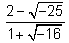Solution:QUESTION: 2

### Express the following in standard form :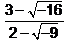Solution:

3-4i/2-3i*2+3i/2+3i =6+9i-8i+12/13=18/13+i/13

QUESTION: 3

### Find the real numbers x and y such that : (x + iy)(3 + 2i) = 1 + i

Solution: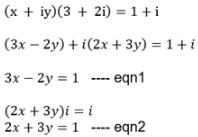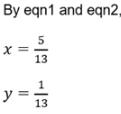QUESTION: 4

Express the following in standard form : (2 – √3i) (2 + √3i) + 2 – 4i

Solution:

Given: (2−√3i)(2+√3i) + 2 − 4i​

(2−√3i)(2+√3i) = 7

⇒ 7 + 2 - 4i

⇒ 9 - 4i

QUESTION: 5

Find the reciprocal (or multiplicative inverse) of -2 + 5i

Solution:

-2+5i
multiplicative inverse of -2+5i is
1/(-2+5i)
=1/-2+5i *-2-5i/-2-5i
=-2-5i/(-2)^2 -(5i)^2
=-2-5i/4-(-25)
=-2-5i/4+25
=-2-5i/29
=-2/29 -5i/29

QUESTION: 6

Find the real numbers x and y such that : (x + iy)(3+2i) = 1 + i

Solution:

(x + iy) (3 + 2i)
= 3x + 2xi + 3iy + 3i*y = 1+i
= 3x-2y + i(2x+3y) = 1+i
= 3x-2y-1 = 0 ; 2x + 3y -1 = 0
on equating real and imaginary parts on both sides
on solving two equations
x= 5/13 ; y = 1/13

QUESTION: 7

Write in the simplest form: (i)-997

Solution:

I^-997= 1/i^997, 1/(i^4)^249 × i, since i^4 = 1, i^4/i= i^3= -i

QUESTION: 8

Express the following in standard form : (8 - 4i) - (-2 - 3i) + (-10 + 3i)

Solution:

(8-4i)-(-2-3i)+(-10+3i)
=>8-4i+2+3i-10+3i
=>8+2-10-4i+3i+3i =>0+2i

QUESTION: 9

Express the following in standard form : (2-3i)2

Solution:

(2-3i)2 = 4 + 9 (i)2 - 2.2.3i

= 4 - 9 - 12i          (because i2 = -1)

= - 5 - 12 i

QUESTION: 10

Find the reciprocal (or multiplicative inverse) of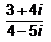Solution: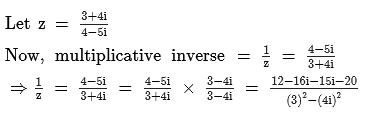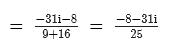QUESTION: 11

Express the following in standard form : i20 + (1 - 2i)3

Solution:

Given, i20 + (1 - 2i)3

We knoe that i = √-1

i2 = -1

Now put the values in given equation

= i20 + (1 - 2i)3

= ( i2)10 + { 1 - 8i3 - 6i + 12i}

= 1 +1 - 8i3 - 6i + 12i2

=1 +1 - 8i2.i1 - 6i + 12i2

=1 + 1 + 8i - 6i -12

=  -10 + 2i

QUESTION: 12

Imaginary part of −i(3i + 2) is

Solution:

(-i)(3i) +2(-i) =-3(i^2)-2i =-3(-1)-2i =3-2i since i=√-1 =3+(-2)i comparing with a+bi,we get b=(-2)

QUESTION: 13

For a complex number a+ib, a-ib is called its

Solution:

This is called conjugate of complex no... z=a+ib. conjugate of z=a-ib - sign are put before i

QUESTION: 14

Express the following in standard form :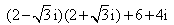Solution: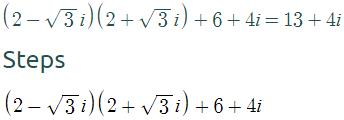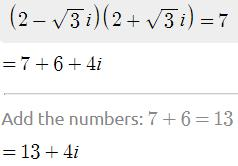QUESTION: 15

The multiplicative inverse of 3 – 4i is

Solution:

Complete answers is in 3 steps: 1. conjugate=3+4i 2. Modulus=√3^2 + 4^2 =5 3. Multiplicative inverse = conjugate/square of modulus =3+4i/5^2 =3+4i/25